• 1中提出用IPW构造出真实CATE的pseudo-label，用于计算评估指标
• 2中提出采用R-learner的损失函数作为评估指标。
• 更多的参考3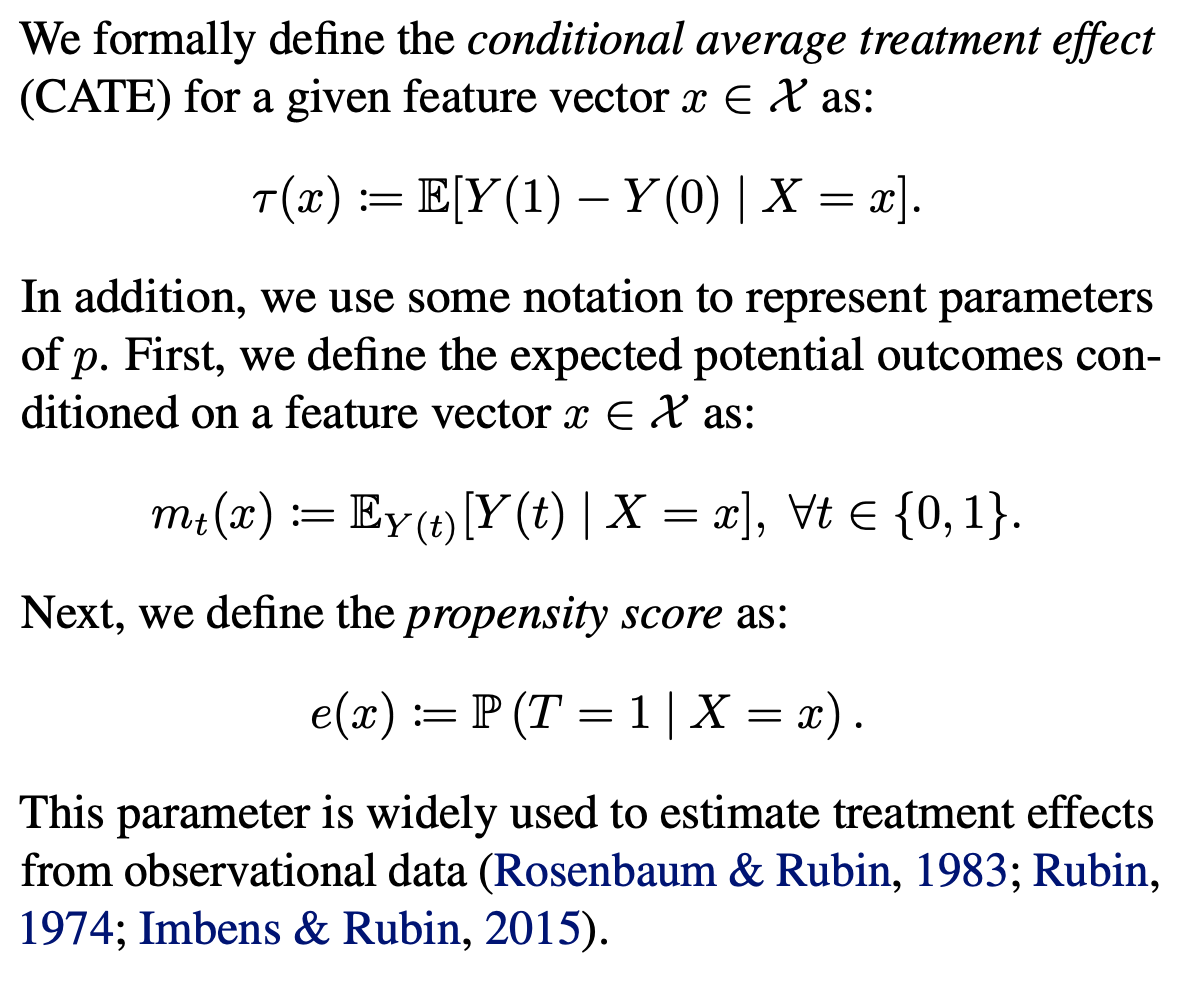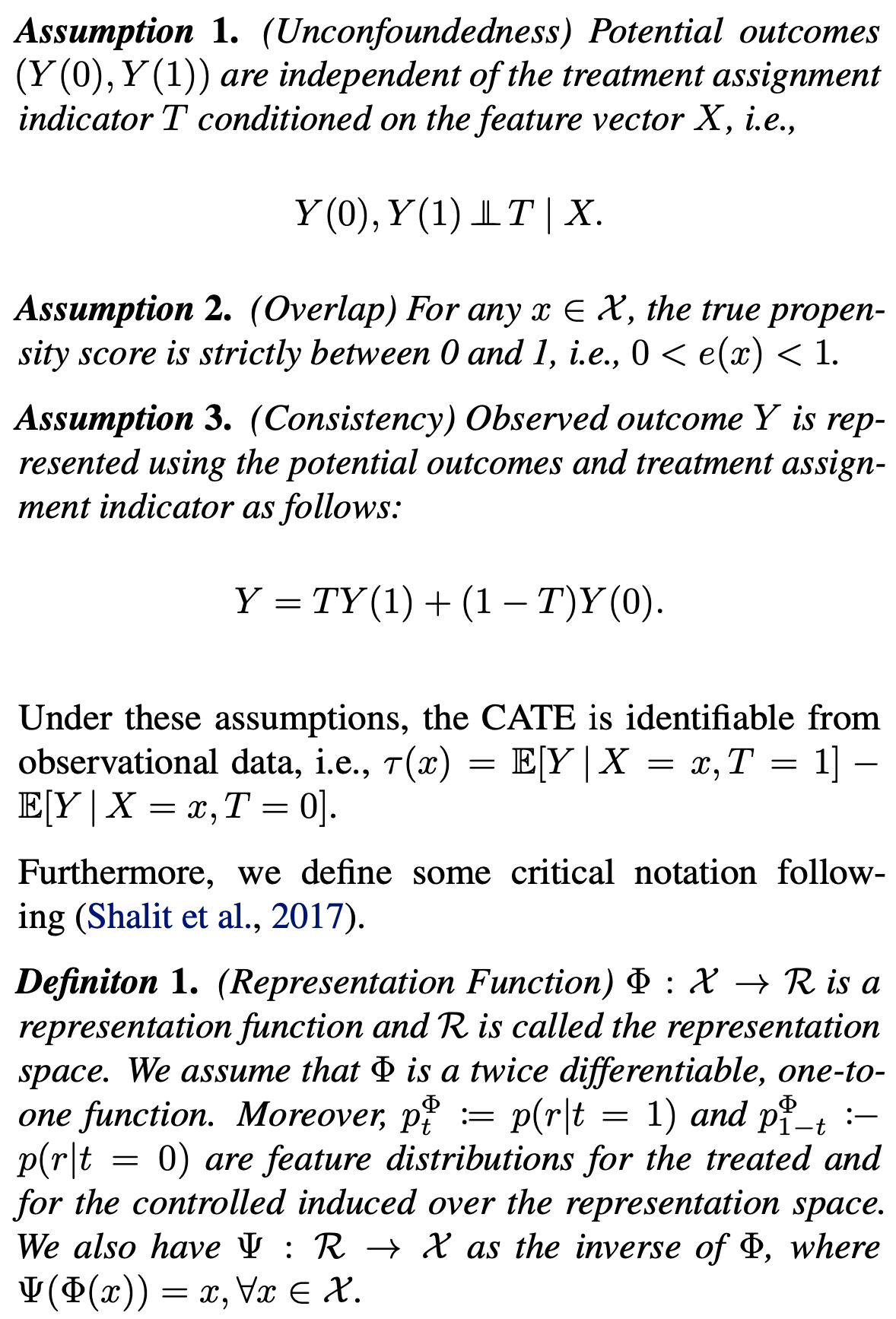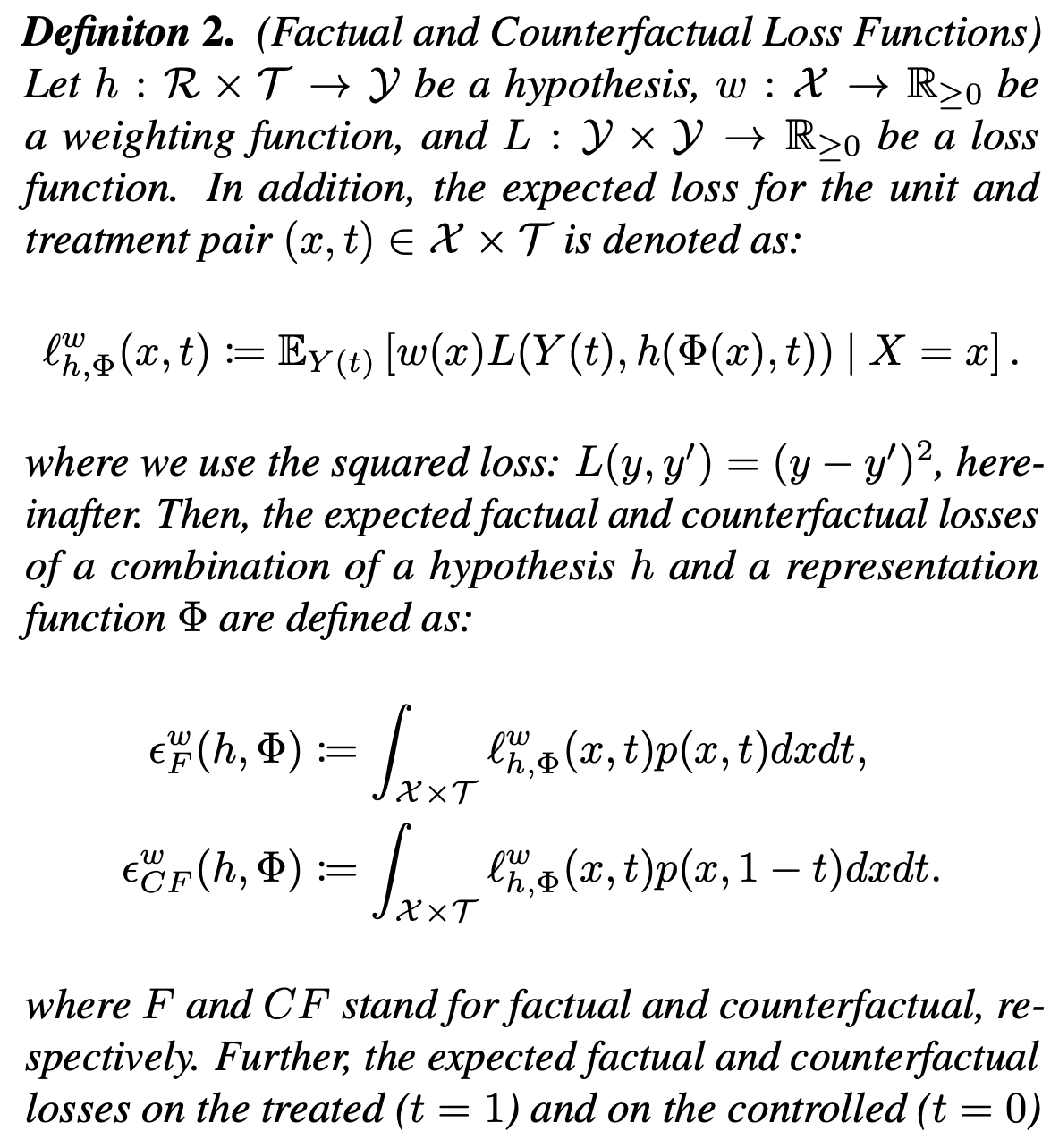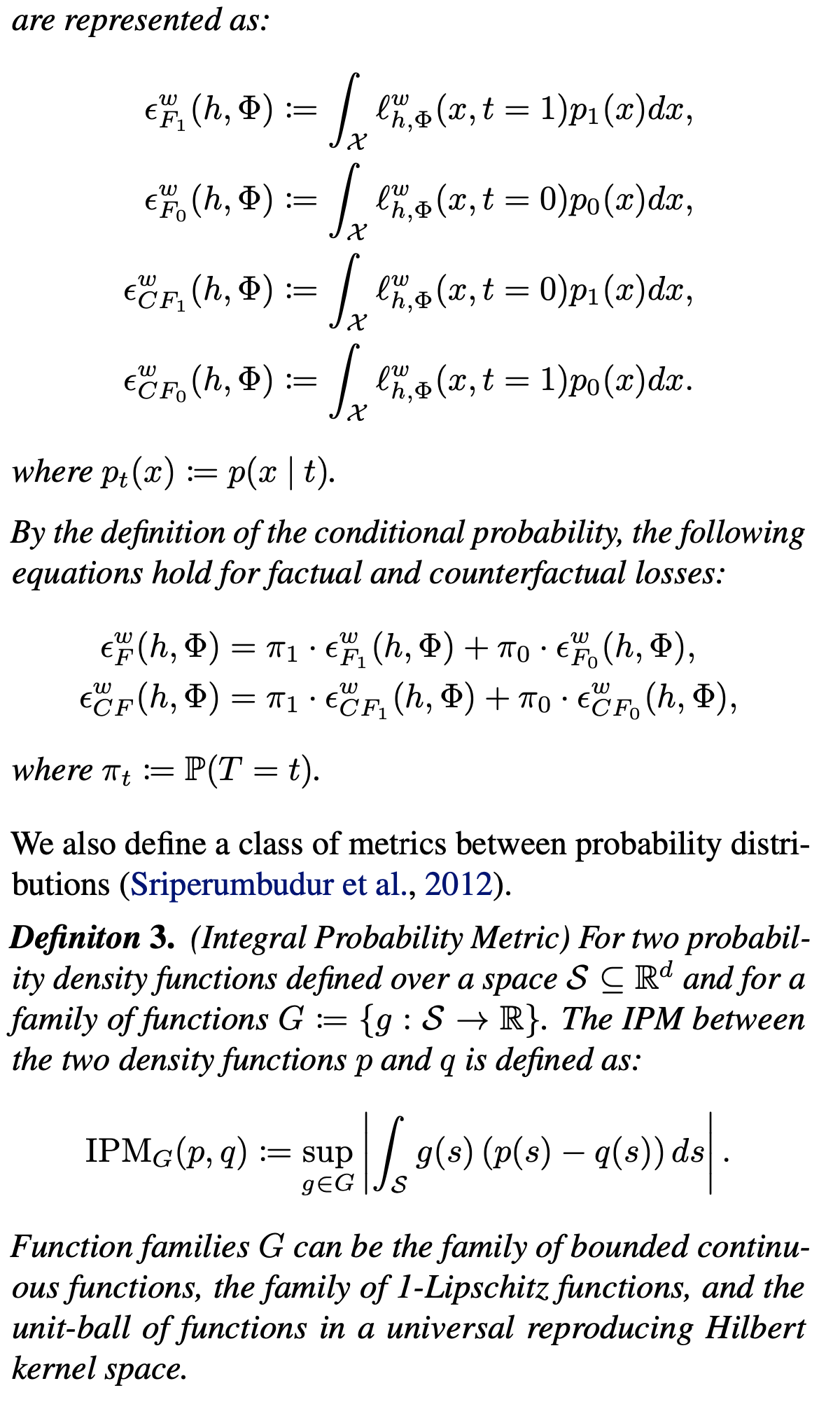\begin{aligned} {\cal{R}}{true}(\hat{\tau})&={\Bbb{E}}[L(\tau(X),\hat{\tau}(X))] \ &={\Bbb{E}}_X[(\tau(X),\hat{\tau}(X))^2] \end{aligned}

$$\cal{R}{true}(\hat{\tau})\leq\cal{R}{true}(\hat{\tau}’)\Rightarrow \hat{\cal{R}}(\hat{\tau})\leq\hat{\cal{R}}(\hat{\tau}’),\forall\hat{\tau},\hat{\tau}’\in\cal{M}$$

$$\hat{\cal{R}}(\hat{\tau})=\frac{1}{n}\sum^n_{i=1}(\tilde{\tau}(X_i,T_i,Y_i)-\hat{\tau}(X_i))^2$$

$$\Bbb{E}[\hat{\cal{R}}(\hat{\tau})]={\cal{R}}{true}(\hat{\tau})+\Bbb{E}[(\tau(X)-{\tilde{\tau}}(X,T,Y))^2]$$

$${\Bbb{E}[\hat{\cal{R}}(\hat{\tau}_1)]} - {\Bbb{E}[\hat{\cal{R}}(\hat{\tau}_2)]} = {\cal{R}} {true}(\hat{\tau}1) - {\cal{R}}{true}(\hat{\tau}_2)$$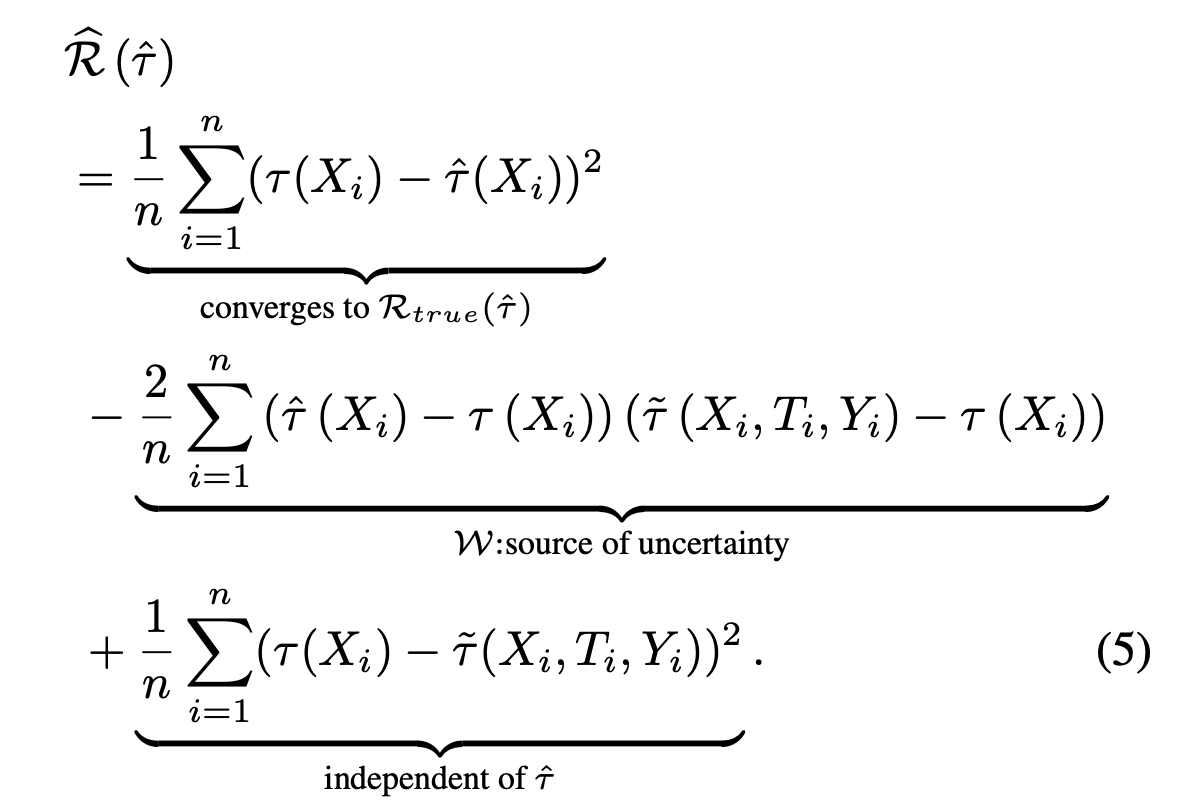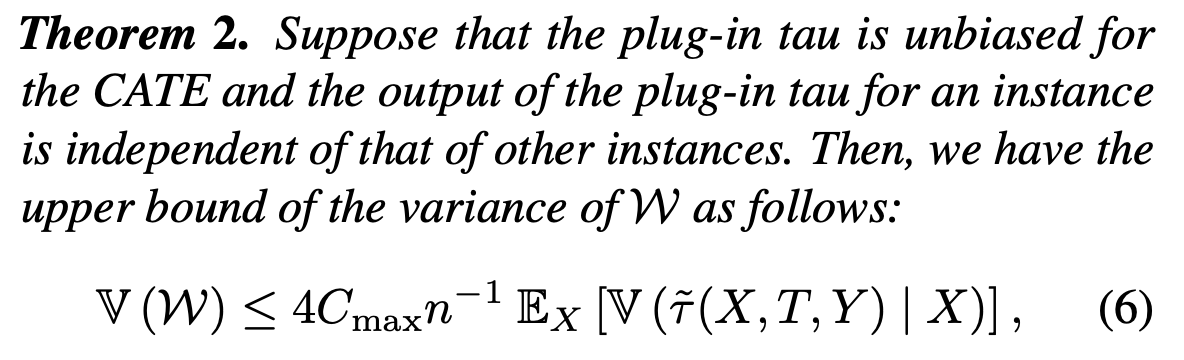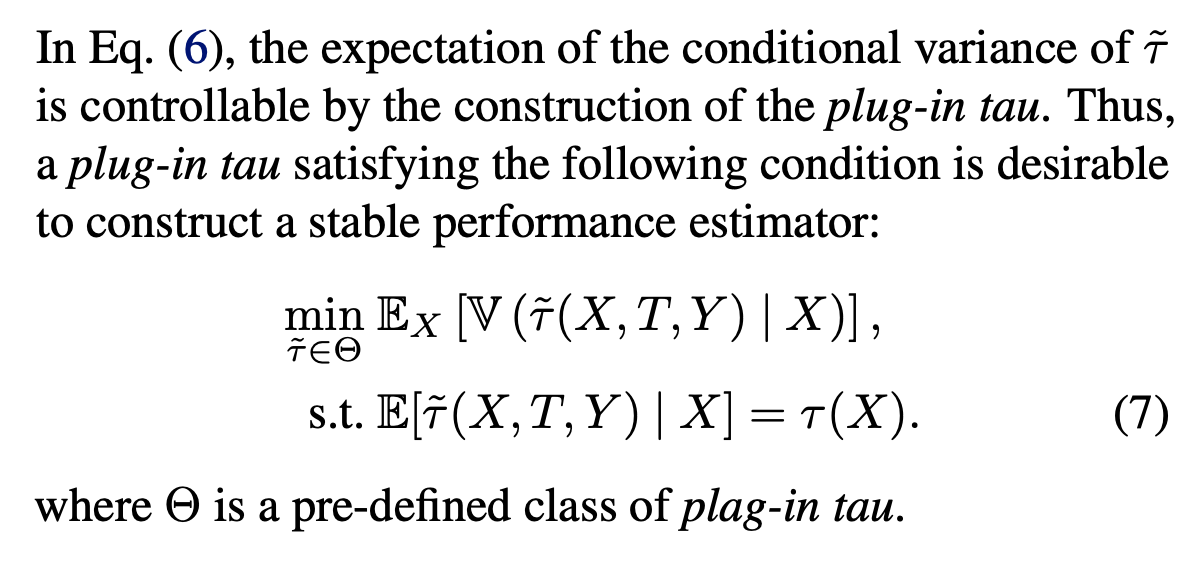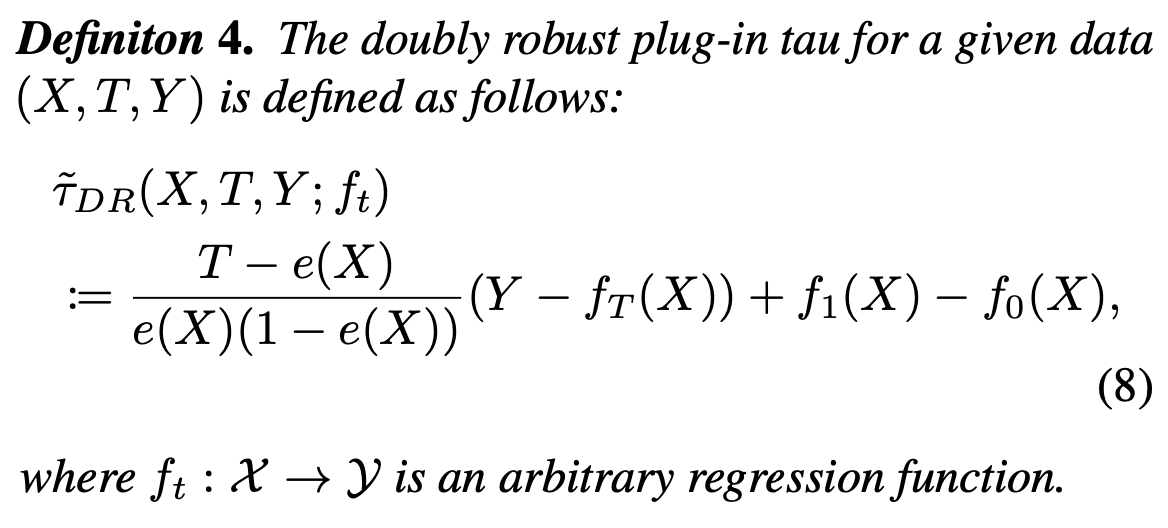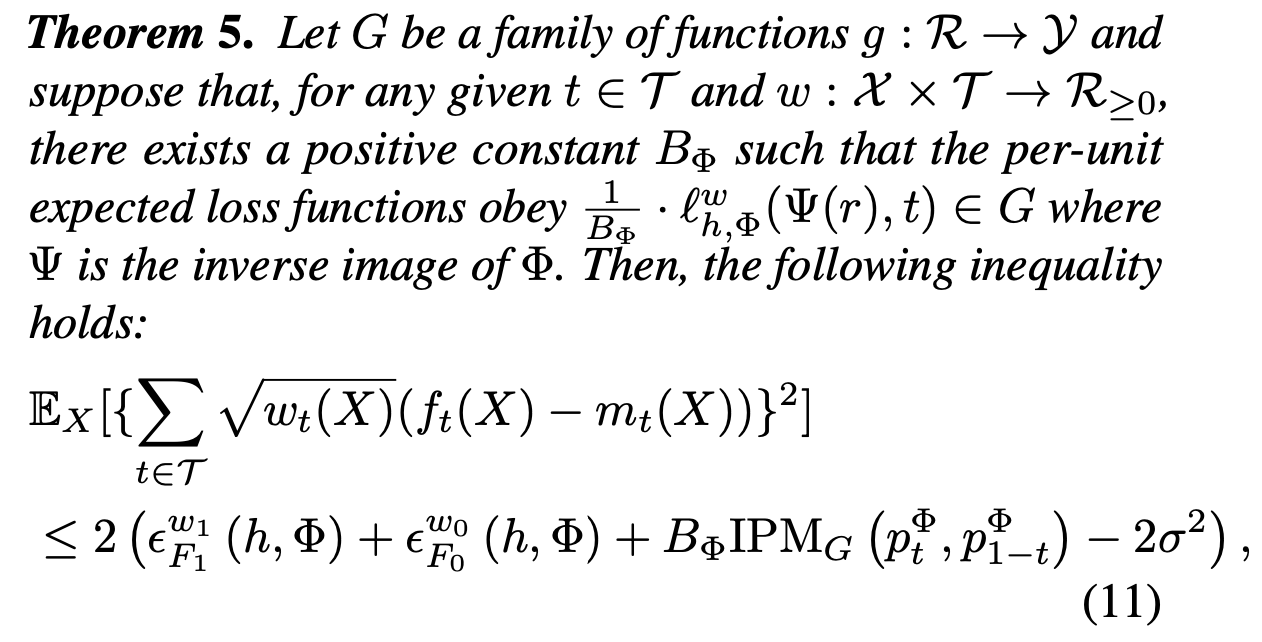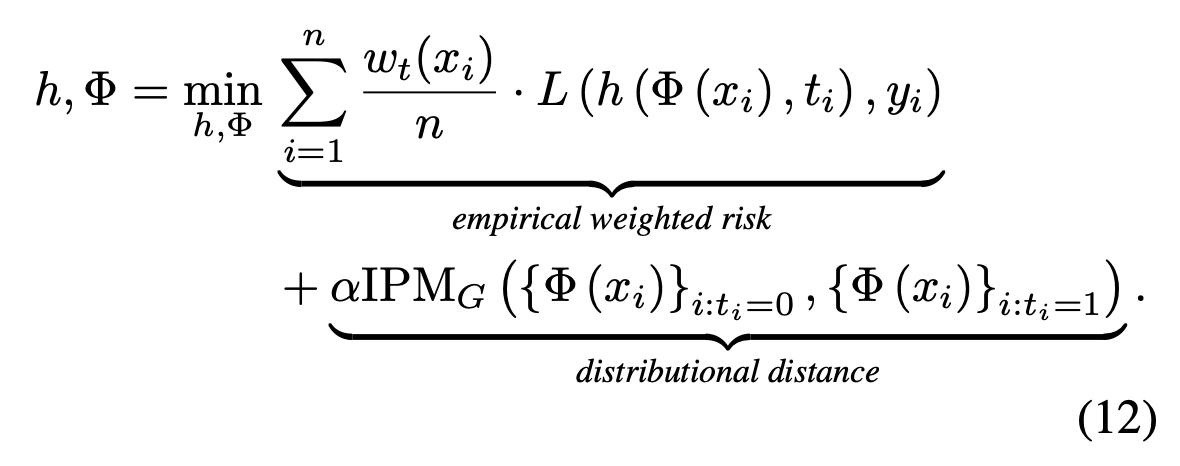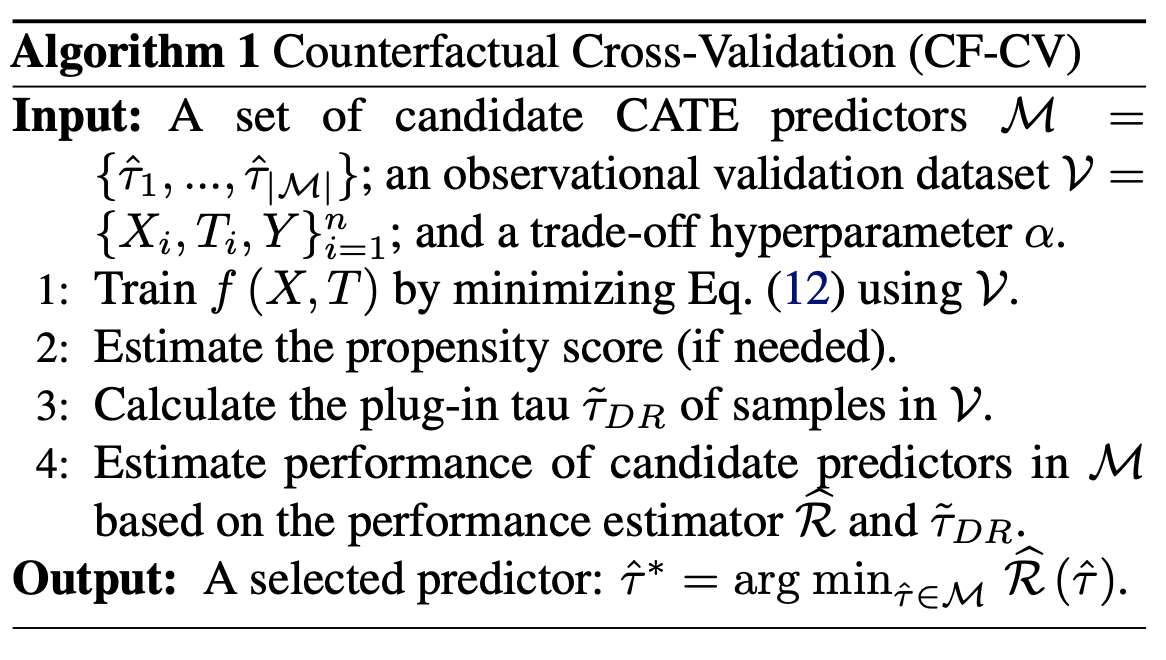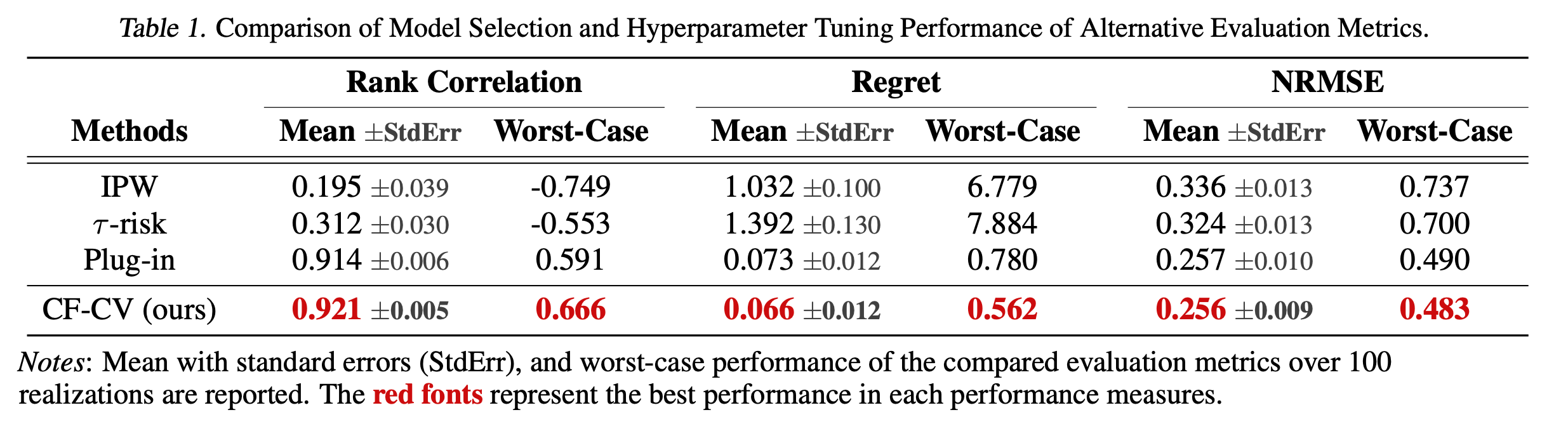# reference

1. Gutierrez, P. and Ge ́rardy, J.-Y. Causal inference and uplift modelling: A review of the literature. In *International Conference on Predictive Applications and APIs*, pp. 1– 13, 2017.
2. Schuler, A., Baiocchi, M., Tibshirani, R., and Shah, N. A comparison of methods for model selection when es- timating individual treatment effects. *arXiv preprint arXiv:1804.05146*, 2018.
1. Schuler, A., Baiocchi, M., Tibshirani, R., and Shah, N. A comparison of methods for model selection when es- timating individual treatment effects. arXiv preprint arXiv:1804.05146, 2018.